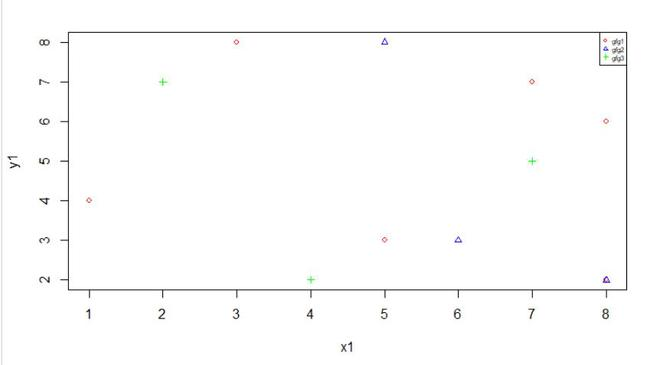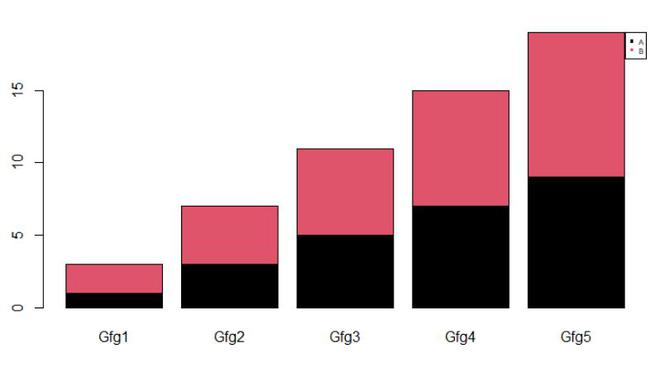# Reduce size of legend area using ggplot in R

• Last Updated : 30 May, 2021

In this article, we will are going to see how to change the size of the legend in the plot in the R programming language. We will change legend size of the plot using cex argument of legend() function.

In this approach to change the legend size of the plot, the user needs to use the cex argument of the legend function and specify its value with the user requirement, the values of cex greater than 1 will increase the legend size in the plot and the value of cex less than 1 will decrease the size of the legend in the plot.

cex: This is a number indicating the amount by which plotting text and symbols should be scaled relative to the default. 1=default, 1.5 is 50% larger, 0.5 is 50% smaller, etc.

Example1: In this example, we will be decreasing the size of the legend of the plot using the cex parameter as 0.5 in the legend() function of the R programming language.

## R

 `x1 <- ``c``(1, 8, 5, 3, 8, 7)                ``y1 <- ``c``(4, 6, 3, 8, 2, 7)`` ` `plot``(x1, y1, cex = .8, pch = 1,``     ``col = ``"red"``)`` ` `x2<-``c``(4, 5, 8, 6, 4)``y2<-``c``(9, 8, 2, 3, 1)``x3<-``c``(2, 1, 6, 7, 4)``y3<-``c``(7, 9, 1, 5, 2)`` ` `points``(x2, y2, cex = .8, pch = 2, col = ``"blue"``)``points``(x3, y3, cex = .8, pch = 3, col = ``"green"``)`` ` `legend``(``"topright"``, ``c``(``"gfg1"``, ``"gfg2"``, ``"gfg3"``),``       ``cex = 0.5, col = ``c``(``"red"``, ``"blue"``, ``"green"``),``       ``pch = ``c``(1, 2, 3))`

Output:Example 2: In this example, we will be decreasing the size of the legend of the plot using the cex parameter as 0.5 in the legend() function of the R programming language.

## R

 `gfg_data <- ``matrix``(``c``(1, 2, 3, 4, 5, 6, 7, 8, 9, 10), ``                   ``ncol = 5)    ``colnames``(gfg_data) <- ``paste0``(``"Gfg"``, 1:5)``rownames``(gfg_data) <- ``c``(``'A'``,``'B'``)`` ` `gfg_data`` ` `barplot``(gfg_data,``        ``col = 1 : ``nrow``(gfg_data))`` ` `legend``(``"topright"``,``       ``legend = ``rownames``(gfg_data),``       ``pch = 15,``       ``col = 1 : ``nrow``(gfg_data), cex = 0.5)`

Output:My Personal Notes arrow_drop_up